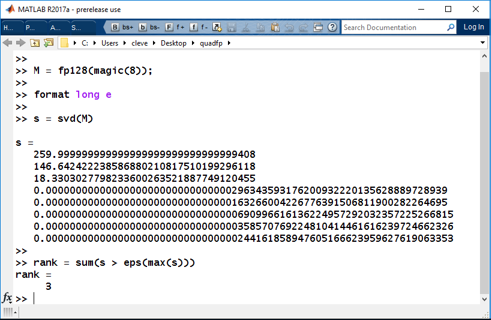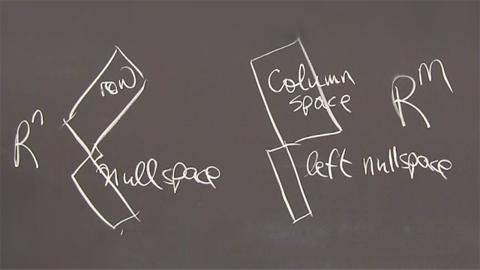# Cleve’s Corner: Cleve Moler on Mathematics and ComputingScientific computing, math & more

Posts 11 - 14 of 14

# Quadruple Precision, 128-bit Floating Point Arithmetic9The floating point arithmetic format that occupies 128 bits of storage is known as binary128 or quadruple precision. This blog post describes an implementation of quadruple precision programmed entirely in the MATLAB language.... 더 읽어보기 >>

# “Half Precision” 16-bit Floating Point Arithmetic5The floating point arithmetic format that requires only 16 bits of storage is becoming increasingly popular. Also known as half precision or binary16, the format is useful when memory is a scarce resource.... 더 읽어보기 >>

# Four Fundamental Subspaces of Linear Algebra, Corrected(Please replace the erroneous posting from yesterday, Nov. 28, with this corrected version.)... 더 읽어보기 >>

# Four Fundamental Subspaces of Linear Algebra3Here is a very short course in Linear Algebra. The Singular Value Decomposition provides a natural basis for Gil Strang's Four Fundamental Subspaces.... 더 읽어보기 >>

Posts 11 - 14 of 14